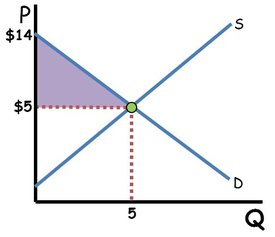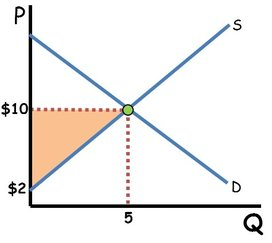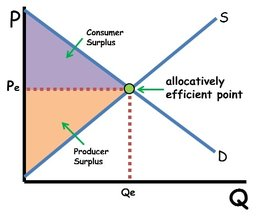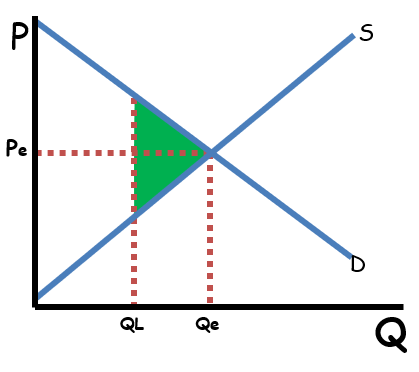### ReviewEcon.com

AP, IB, and College Microeconomic and Macroeconomic Principles

# Consumer Surplus, Producer Surplus, and Deadweight Loss

9/5/2018 Jacob Reed
What is consumer surplus?

Consumer surplus is the difference between what a consumer is willing to pay and what they actually pay. If you go to the mall to purchase a new pair of shoes, and you are willing to pay \$75 but you arrive to see them on sale for \$50, you would surely buy the shoes since the marginal benefit of owning them (\$75) far exceeds the marginal cost of buying them. In this example, your consumer surplus would be \$25.How do you find consumer surplus in a market?

The demand curve in a competitive free market represents the price consumers are willing to pay for each quantity of a product. The equilibrium price is how much consumers will actually pay for that product. The consumer surplus can be found by forming a triangle from the equilibrium price on the Y axis, to the equilibrium point where supply and demand intersect, and where the demand curve hits the Y axis. This triangle is the consumer surplus. To calculate the value of the consumer surplus, find the area of the triangle (½ base times height). In the graph above the consumer surplus would be \$22.50(\$9 x 5 x ½).

What is producer surplus?

Producer surplus is the difference between the lowest price producers are willing to accept and the price they actually receive. If you are willing to provide babysitting service for a family friend when the pay is at least \$10 an hour, but you are offered \$15 per hour instead, you would take the job and your producer surplus would be \$5 per hour. Generally, the lowest price producers are willing to accept is equal to their marginal cost of production.How do you find producer surplus in a market?

In a market, the supply curve represents the minimum price producers are willing to accept for different quantities produced. The equilibrium price is what producers receive for that service. The producer surplus can be found by forming a triangle from the equilibrium price on the Y axis, to the equilibrium point where supply and demand intersect, to where the supply curve hits the Y axis. This triangle is the producer surplus. To calculate the value of the producer surplus, find the area of the triangle (½ base times height). In the graph above, the producer surplus would be \$20 (\$8 x 5 x ½).

What is economic surplus?

Economic surplus is the sum of both consumer and producer surplus. A market is considered allocatively efficient when economic surplus is maximized.

Note: Any tax revenue (see excise taxes or the excise tax graph below) would also be part of economic surplus.Deadweight loss (sometimes called efficiency loss) occurs when economic surplus is not maximized. Deadweight loss is a decrease in efficiency caused by a market not reaching a competitive equilibrium. It can be caused by price floors, price ceilingsexcise taxesnoncompetitive markets, or negative and positive externalities. Deadweight loss is generally illustrated on a graph with a triangle formed by the 3 points of the allocatively efficient point (where the marginal benefit to society equals to the marginal cost to society), the marginal benefit to society for the current quantity (the demand curve if there are no externalities), and the marginal cost to society for the current quantity (the supply curve if there are no externalities). If, for example, this market was producing at QL, the allocatively efficient quantity would be Qe and the green triangle would be deadweight loss. The value of the deadweight loss can also be calculated by finding the area of the triangle (½ base times height).Here are some other examples of producer surplus, consumer surplus and deadweight loss that you will see on other content reviews: